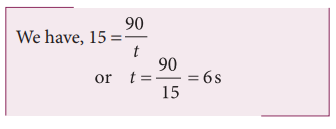Home | | Physics 11th std | Solved Example Problems for Relative Velocity in One and Two Dimensional Motion

# Solved Example Problems for Relative Velocity in One and Two Dimensional Motion

Physics : Kinematics : Relative Velocity in One and Two Dimensional Motion

Solved Example Problems for Relative Velocity in One and Two Dimensional Motion

Example 2.26

Suppose two cars A and B are moving with uniform velocities with respect to ground along parallel tracks and in the same direction. Let the velocities of A and B be 35 km h-1 due east and 40 km h-1 due east respectively. What is the relative velocity of car B with respect to A?Solution

The relative velocity of B with respect to A,due east

Similarly, the relative velocity of A with respect to B i.e.,due west.

Example 2.27

Suppose two trains A and B are moving with uniform velocities along parallel tracks but in opposite directions. Let the velocity of train A be 40 km h-1 due east and that of train B be 40 km h-1 due west. Calculate the relative velocities of the trains

Solution

Relative velocity of A with respect to B, vAB = 80 km h−1 due east

Thus to a passenger in train B, the train A will appear to move east with a velocity  of 80 km h−1

The relative velocity of B with respect to A, VBA = 80 km h−1  due west

To a passenger in train A, the train B will appear to move westwards with a velocity of 80 km h−1

Example 2.28

Consider two trains A and B moving along parallel tracks with the same velocity in the same direction. Let the velocity of each train be 50 km h-1 due east. Calculate the relative velocities of the trains

SolutionSimilarly, relative velocity of A with respect to B i.e., vAB is also zero.

Thus each train will appear to be at rest with respect to the other.

Example 2.29

How long will a boy sitting near the window of a train travelling at 36 km h-1 see a train passing by in the opposite direction with a speed of 18 km h-1. The length of the slow-moving train is 90 m.

Solution

The relative velocity of the slow-moving train with respect to the boy is = (36 + 18) km h-1 = 54 km h-1 = = 54 × 5/18 ms-1 = 15 m s-1

Since the boy will watch the full length of the other train, to find the time taken to watch the full train:Example 2.30

A swimmer’s speed in the direction of flow of a river is 12 km h-1. Against the direction of flow of the river the swimmer’s speed is 6 km h-1. Calculate the swimmer’s speed in still water and the velocity of the river flow.

Solution

Let vs and vr , represent the velocities of the swimmer and river respectively with respect to ground.When the river flow and swimmer move in the same direction, the net velocity of swimmer is 12 km p h-1.

Study Material, Lecturing Notes, Assignment, Reference, Wiki description explanation, brief detail
11th Physics : UNIT 2 : Kinematics : Solved Example Problems for Relative Velocity in One and Two Dimensional Motion |